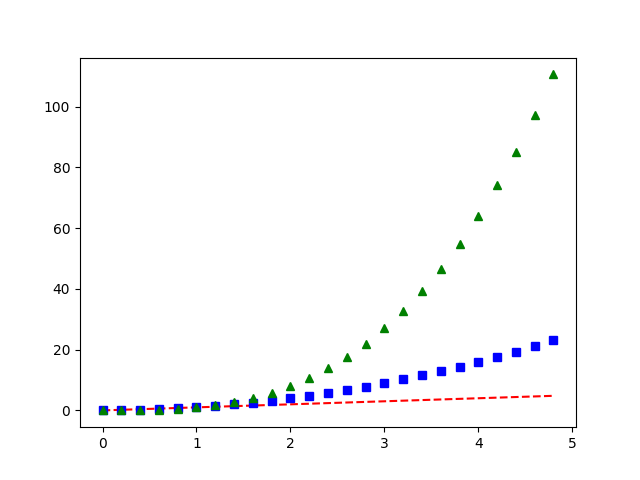Travis-CI:# Pyplot Three¶```import numpy as np
import matplotlib.pyplot as plt

# evenly sampled time at 200ms intervals
t = np.arange(0., 5., 0.2)

# red dashes, blue squares and green triangles
plt.plot(t, t, 'r--', t, t**2, 'bs', t, t**3, 'g^')
plt.show()
```

Total running time of the script: ( 0 minutes 0.019 seconds)

Gallery generated by Sphinx-Gallery